# Recursion CS240CS 341 What is recursion a function

• Slides: 12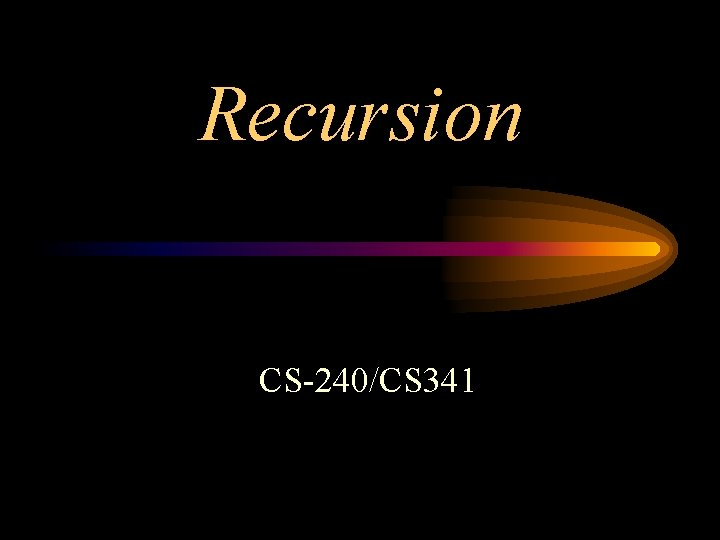Recursion CS-240/CS 341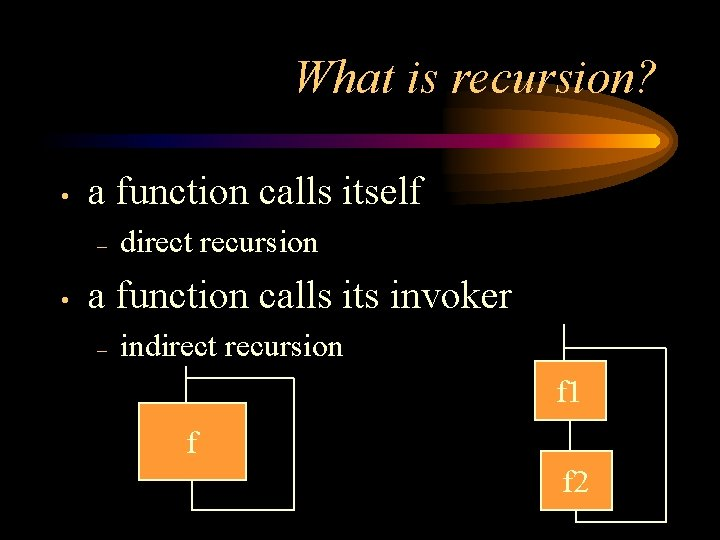What is recursion? • a function calls itself – • direct recursion a function calls its invoker – indirect recursion f 1 f f 2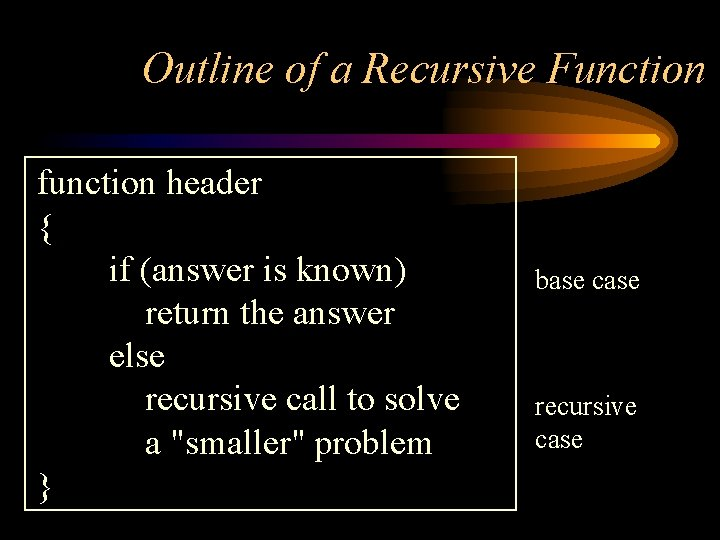Outline of a Recursive Function function header { if (answer is known) return the answer else recursive call to solve a "smaller" problem } base case recursive caseFactorial (n) - recursive Factorial (n) = n * Factorial (n-1) Factorial (0) = 1 base case (for n > 0) long factorial (int n) { if (n == 0) return 1; else return n * factorial (n-1); }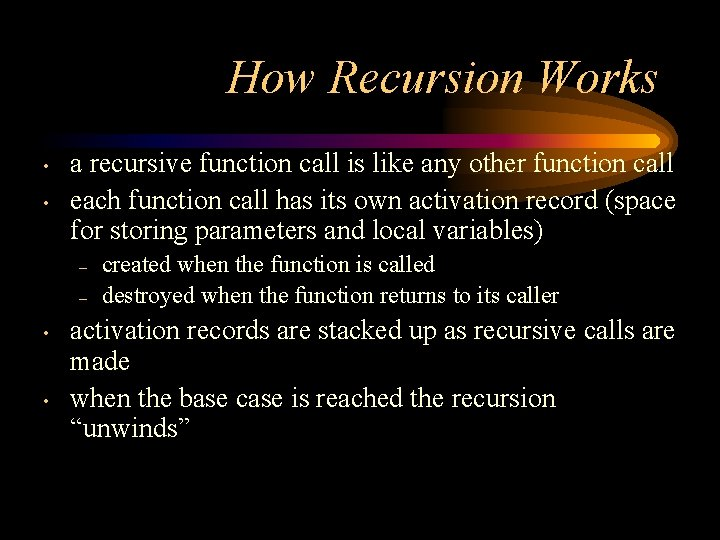How Recursion Works • • a recursive function call is like any other function call each function call has its own activation record (space for storing parameters and local variables) – – • • created when the function is called destroyed when the function returns to its caller activation records are stacked up as recursive calls are made when the base case is reached the recursion “unwinds”Recursive Call Tree long factorial (int n) { if (n == 0) return 1; else return n * factorial (n-1); } What happens with factorial (-2)? Rec. Fact (4) 24 4 6 3 2 2 1 1 0 1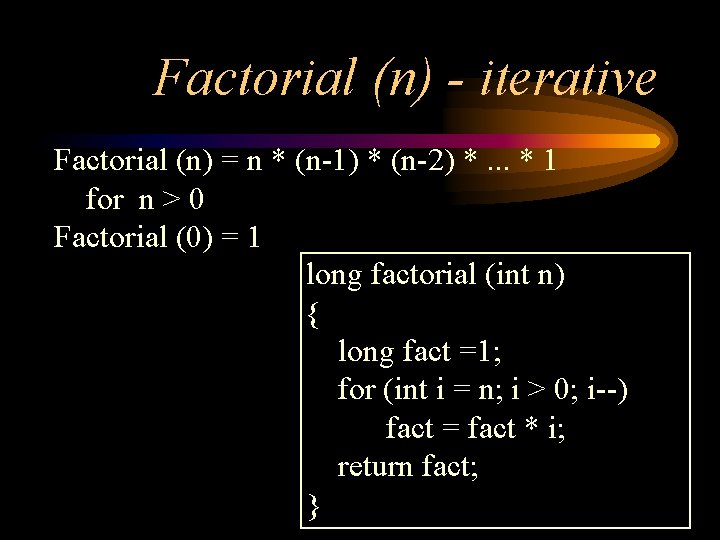Factorial (n) - iterative Factorial (n) = n * (n-1) * (n-2) *. . . * 1 for n > 0 Factorial (0) = 1 long factorial (int n) { long fact =1; for (int i = n; i > 0; i--) fact = fact * i; return fact; }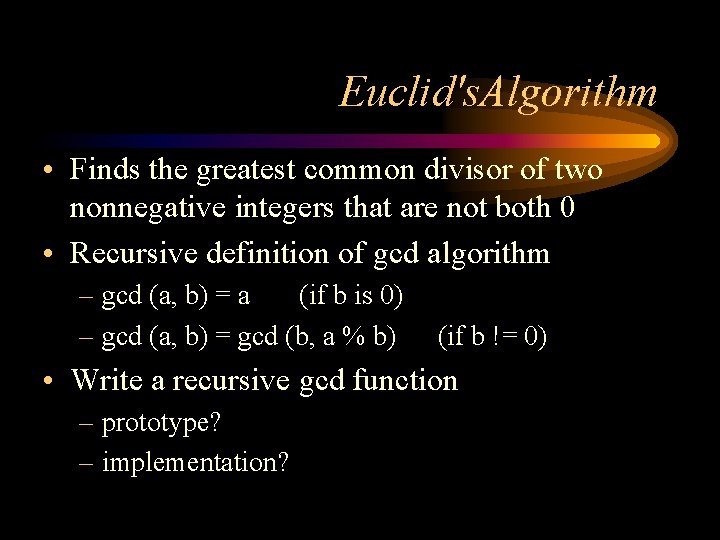Euclid's. Algorithm • Finds the greatest common divisor of two nonnegative integers that are not both 0 • Recursive definition of gcd algorithm – gcd (a, b) = a (if b is 0) – gcd (a, b) = gcd (b, a % b) (if b != 0) • Write a recursive gcd function – prototype? – implementation?Tracing gcd (54, 30) int gcd (int a, int b) { if (b == 0) return a; else return gcd (b, a % b); } 54, 30 30, 24 24, 6 6, 0 6 6Iterative gcd? int gcd (int a, int b) { int temp; while (b != 0) { temp = b; b = a % b; a = temp; } return a; }Another recursive definition Fibonacci numbers are the sequence of integers: 0, 1, 1, 2, 3, 5, 8, 13, 21, 34, 55, …. fib (0) = 0 fib (1) = 1 fib (n) = fib (n – 1) + fib (n – 2) (for n >= 2) int fib (int n) { if (n <= 1) return n; else return fib (n – 1) + fib (n – 2); }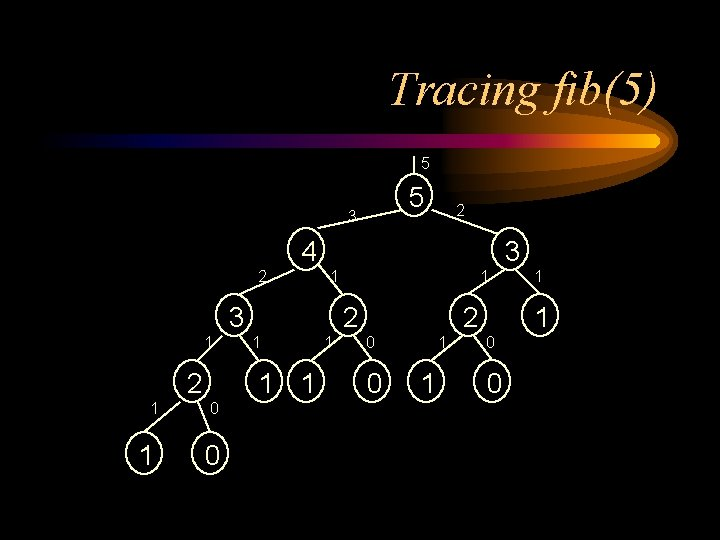Tracing fib(5) 5 5 3 2 4 2 3 1 2 2 1 1 0 1 1 2 0 0 1 1 0 0